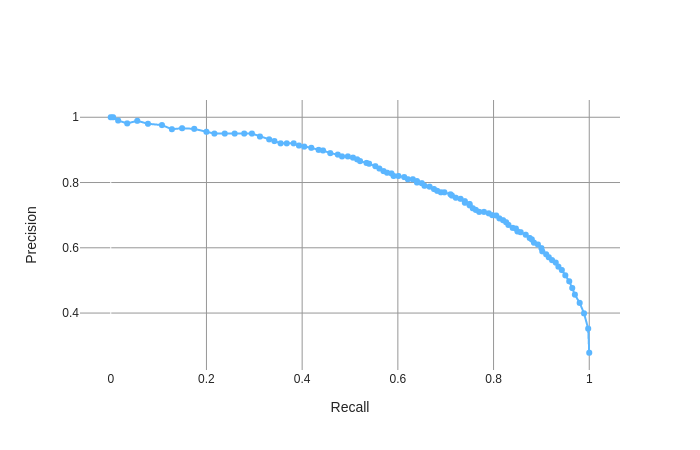Search

# Precision-Recall Curve

Precision-Recall Curve is a graphic way to evaluate the model performance and trade-offs under different working points.

Precision is a ratio of the number of true positives divided by the sum of positive prediction according to the model (both correct and wrong). It describes how gooda model is at predicting the positive class. Precision is referred to as the positive predictive value. Intuitively, precision tells us how much should we trust an alarm (positive prediction) raised by the model.

Recall (also know as sensitivity) is calculated as the ratio between the number of true positives divided by the all the positives in the data. The recall tells us how likely the model is at correctly alarming a positive sample in the data.

Reviewing both precision and recall is useful in cases where the predicted classes are not balanced. For example, In fraud analysis true frauds consists less than 0.1% of the data. A model that will predict "not fraud" for every sample will be accurate 99.9% of the time, but its recall will be 0%.A precision-recall curve is a plot of the precision (y-axis) and the recall (x-axis) using different working points. The user can choose the desired trade off between precision and recall by choosing the corresponding working point on the precision-recall curve.

See All